# What is the effect of current harmonics?

Definition

The theory of AC (Alternative Current) rotating machines considers sinusoidal supply currents, but in reality it is usually composed of an addition of multiple harmonics. For a given phase, the current waveform can be written as:With:

•the amplitude of the harmonic
•the frequency of the harmonic
•the phase of the harmonicFigure 1: Waveform and spectrum of a theoretical currentFigure 2: Waveform and spectrum of a more realistic current

Different physical phenomena lead to the creation of these harmonics:

• Supply voltage harmonics due to Pulse Width Modulation (PWM) or six-step operation
• Control harmonics induced on purpose to reduce magnetic noise (Harmonic Current Injection) or to increase average torque or efficiency
• Voltage harmonics induced by magnetic flux harmonics (e.g. back emf in PMSM, Rotor Slot Harmonic in IM) due to strong electrical to magnetic coupling
• Voltage harmonics introduced by non-linearities of the inverter or power converter

These sources of harmonics may be found when measuring the current waveforms of an electric machine.

Application to e-NVH

Additional current harmonics create additional flux harmonics, which in turn create additional force harmonics. Current harmonics mainly induce additional magnetic forces of wavenumber 0 and 2p (number of poles). This additional forces can result in additional magnetic noise and vibration, especially when they resonate with a structural mode of the electric drive.

Application to Manatee

In Manatee e-NVH software, current harmonics can be defined as an input of a current-driven simulation. Sensitivity study and multi-objective optimization can be run on 6fe, 12fe and 18fe current harmonics as well. Finally, a specific Harmonic Current Injection environment dedicated to noise reduction is provided. In Manatee the current harmonics are defined by an amplitude and a phase relative to the fundamental. The current is computed with the following equation: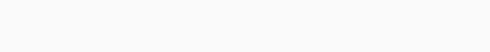With :

•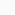the amplitude of the fundamental computed via Id and Iq
•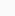the frequency of the fundamental (stator current electrical frequency)
•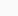the phase of the fundamental
•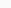the rank of the harmonic
•the amplitude of the harmonic with the rank n
•the relative phase of the harmonic with the rank n
•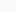the number of harmonics defined by the user